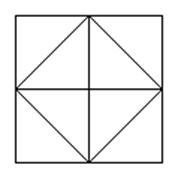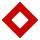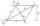# Rhombus IV

Calculate the length of the diagonals of the rhombus, whose lengths are in the ratio 1: 2 and a rhombus side is 35 cm.

u =  31.305 cm
v =  62.61 cm

### Step-by-step explanation:We will be pleased if You send us any improvements to this math problem. Thank you!Tips to related online calculators
Pythagorean theorem is the base for the right triangle calculator.
See also our trigonometric triangle calculator.

#### You need to know the following knowledge to solve this word math problem:

We encourage you to watch this tutorial video on this math problem:

## Related math problems and questions:

• RhombusCalculate the perimeter and area of ​​a rhombus whose diagonals are 39 cm and 51 cm long.
• Rhombus and diagonalsThe lengths of the diamond diagonals are e = 48cm, f = 20cm. Calculate the length of its sides.
• Rhombus and inscribed circleIt is given a rhombus with side a = 6 cm and the radius of the inscribed circle r = 2 cm. Calculate the length of its two diagonals.
• Two diagonalsThe diagonals of the diamond EFGH have lengths in the ratio 1: 2. What is the circumference of a rhombus if the longer of the diagonals is 8 cm long?
• DiagonalsA diagonal of a rhombus is 20 cm long. If it's one side is 26 cm, find the length of the other diagonal.
• DiamondSide length of diamond is 35 cm and the length of the diagonal is 56 cm. Calculate the height and length of the second diagonal.
• Diamond diagonalsCalculate the diamonds' diagonals lengths if the diamond area is 156 cm square, and the side length is 13 cm.
• Cuboid face diagonalsThe lengths of the cuboid edges are in the ratio 1: 2: 3. Will the lengths of its diagonals be the same ratio? The cuboid has dimensions of 5 cm, 10 cm, and 15 cm. Calculate the size of the wall diagonals of this cuboid.
• DiagonalsGiven a rhombus ABCD with a diagonalsl length of 8 cm and 12 cm. Calculate the side length and content of the rhombus.
• Diagonals in diamons/rhombusRhombus ABCD has side length AB = 4 cm and a length of one diagonal of 6.4 cm. Calculate the length of the other diagonal.
• Diagonals of the rhombusCalculate height of rhombus whose diagonals are 12 cm and 19 cm.
• RhombusOne angle of a rhombus is 136°, and the shorter diagonal is 8 cm long. Find the length of the longer diagonal and the side of the rhombus.
• RhombusIt is given a rhombus of side length a = 19 cm. Touchpoints of inscribed circle divided his sides into sections a1 = 5 cm and a2 = 14 cm. Calculate the radius r of the circle and the length of the diagonals of the rhombus.
• Diamond sideThe diagonals of the diamond are 18 cm and 14 cm long. Calculate the length of the diamond side.
• Two diagonalsThe rhombus has a side length of 12 cm and a length of one diagonal of 21 cm. What is the length of the second diagonal?
• A rectangle 2A rectangle has a diagonal length of 74cm. Its side lengths are in ratio 5:3. Find its side lengths.
• Right trapezoidThe right trapezoid has bases 3.2 cm and 62 mm long. The shorter leg has a length 0.25 dm. Calculate the lengths of the diagonals and the second leg.